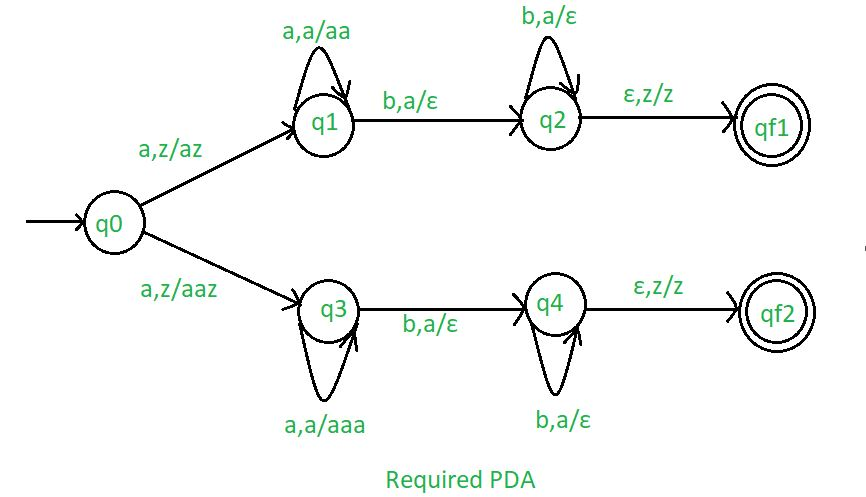# NPDA for accepting the language L = {anb(2n) | n>=1} U {anbn | n>=1}

Prerequisite – Pushdown automata, Pushdown automata acceptance by final state

Problem – Design a non deterministic PDA for accepting the language L = {[Tex]b^{2n}  [/Tex]: n>=1} U {[Tex]b^{n}  [/Tex]: n>=1}, i.e.,

L = {abb, aabbbb, aaabbbbbb, aaaabbbbbbbb, ......}
U {ab, aabb, aaabbb, aaaabbbb, ......} 

In each string, the number of a’s are followed by double number of b’s or the number of a’s are followed by equal number of b’s.

Explanation – Here, we need to maintain the order of a’s and b’s.That is, all the a’s are  coming first and then all the b’s are coming. Thus, we need a stack along with the state diagram. The count of a’s and b’s is maintained by the stack.We will take 2 stack alphabets:= { a, z }

Where,= set of all the stack alphabet z = stack start symbol

Approach used in the construction of PDA – In designing a NPDA, for every ‘a’ comes before ‘b’. If ‘b’ comes then

• For[Tex]b^{n}  [/Tex]: Whenever ‘a’ comes, push it in stack and if ‘a’ comes again then also push it in the stack.
• For[Tex]b^{2n}  [/Tex]: Whenever ‘a’ comes, push ‘a’ two time in stack and if ‘a’ comes again then do the same. When ‘b’ comes (remember b comes after ‘a’) then pop one ‘a’ from the stack each time.

So that the stack becomes empty.If stack is empty then we can say that the string is accepted by the PDA.

Stack transition functions –(q0, a, z)(q1, az)(q0, a, z)(q3, aaz)(q1, a, a)(q1, aa)(q1, b, a)(q2,)(q2, b, a)(q2,)(q2,, z)(qf1, z)(q3 a, a)(q3, aaa)(q3, b, a)(q4,)(q4, b, a)(q4,)(q4,, z)(qf2, z)

Where, q0 = Initial state qf1, qf2 = Final state= indicates pop operationSo, this is our required non deterministic PDA for accepting the language L ={[Tex]b^{2n}  [/Tex]: n>=1} U {[Tex]b^{n}  [/Tex]: n>=1}.